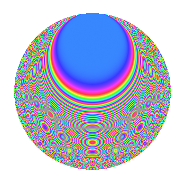# Properties

 Label 4016.2.aLevel 4016 Weight 2 Character orbit a Rep. character $$\chi_{4016}(1,\cdot)$$ Character field $$\Q$$ Dimension 125 Newform subspaces 13 Sturm bound 1008 Trace bound 3

# Related objects

## Defining parameters

 Level: $$N$$ = $$4016 = 2^{4} \cdot 251$$ Weight: $$k$$ = $$2$$ Character orbit: $$[\chi]$$ = 4016.a (trivial) Character field: $$\Q$$ Newform subspaces: $$13$$ Sturm bound: $$1008$$ Trace bound: $$3$$ Distinguishing $$T_p$$: $$3$$

## Dimensions

The following table gives the dimensions of various subspaces of $$M_{2}(\Gamma_0(4016))$$.

Total New Old
Modular forms 510 125 385
Cusp forms 499 125 374
Eisenstein series 11 0 11

The following table gives the dimensions of the cuspidal new subspaces with specified eigenvalues for the Atkin-Lehner operators and the Fricke involution.

$$2$$$$251$$FrickeDim.
$$+$$$$+$$$$+$$$$21$$
$$+$$$$-$$$$-$$$$42$$
$$-$$$$+$$$$-$$$$31$$
$$-$$$$-$$$$+$$$$31$$
Plus space$$+$$$$52$$
Minus space$$-$$$$73$$

## Trace form

 $$125q + 2q^{3} - 2q^{5} + 2q^{7} + 125q^{9} + O(q^{10})$$ $$125q + 2q^{3} - 2q^{5} + 2q^{7} + 125q^{9} - 4q^{11} - 2q^{13} - 4q^{15} + 2q^{17} - 4q^{19} - 8q^{21} - 10q^{23} + 127q^{25} - 4q^{27} - 2q^{29} + 2q^{31} - 2q^{37} + 8q^{39} + 2q^{41} - 4q^{43} - 18q^{45} - 12q^{47} + 133q^{49} + 28q^{51} - 2q^{53} + 8q^{55} + 8q^{57} - 12q^{59} - 2q^{61} + 6q^{63} + 12q^{65} + 2q^{67} - 8q^{69} + 32q^{71} + 18q^{73} - 2q^{75} - 16q^{77} - 10q^{79} + 133q^{81} + 10q^{83} - 12q^{85} + 48q^{87} + 10q^{89} + 56q^{91} - 8q^{93} - 8q^{95} + 2q^{97} + 40q^{99} + O(q^{100})$$

## Decomposition of $$S_{2}^{\mathrm{new}}(\Gamma_0(4016))$$ into newform subspaces

Label Dim. $$A$$ Field CM Traces A-L signs $q$-expansion
$$a_2$$ $$a_3$$ $$a_5$$ $$a_7$$ 2 251
4016.2.a.a $$2$$ $$32.068$$ $$\Q(\sqrt{13})$$ None $$0$$ $$-2$$ $$5$$ $$2$$ $$-$$ $$-$$ $$q-q^{3}+(2+\beta )q^{5}+q^{7}-2q^{9}-3q^{11}+\cdots$$
4016.2.a.b $$2$$ $$32.068$$ $$\Q(\sqrt{5})$$ None $$0$$ $$2$$ $$-3$$ $$4$$ $$-$$ $$+$$ $$q+q^{3}+(-2+\beta )q^{5}+(1+2\beta )q^{7}-2q^{9}+\cdots$$
4016.2.a.c $$4$$ $$32.068$$ 4.4.725.1 None $$0$$ $$2$$ $$-3$$ $$3$$ $$-$$ $$-$$ $$q+\beta _{2}q^{3}+(-1+\beta _{1}+\beta _{2}-\beta _{3})q^{5}+\cdots$$
4016.2.a.d $$5$$ $$32.068$$ 5.5.138917.1 None $$0$$ $$-2$$ $$7$$ $$-1$$ $$-$$ $$+$$ $$q+\beta _{4}q^{3}+(2+\beta _{1}+\beta _{2}-\beta _{3})q^{5}+(\beta _{2}+\cdots)q^{7}+\cdots$$
4016.2.a.e $$5$$ $$32.068$$ 5.5.242773.1 None $$0$$ $$1$$ $$-6$$ $$1$$ $$-$$ $$-$$ $$q+\beta _{1}q^{3}+(-1+\beta _{2})q^{5}+(1-2\beta _{1}+\cdots)q^{7}+\cdots$$
4016.2.a.f $$6$$ $$32.068$$ 6.6.60853001.1 None $$0$$ $$-1$$ $$-1$$ $$-6$$ $$-$$ $$-$$ $$q+\beta _{4}q^{3}+(-1+\beta _{1}-\beta _{3})q^{5}+(-1+\cdots)q^{7}+\cdots$$
4016.2.a.g $$7$$ $$32.068$$ $$\mathbb{Q}[x]/(x^{7} - \cdots)$$ None $$0$$ $$3$$ $$-2$$ $$6$$ $$-$$ $$+$$ $$q+\beta _{1}q^{3}+\beta _{5}q^{5}+(1+\beta _{6})q^{7}+(-1+\cdots)q^{9}+\cdots$$
4016.2.a.h $$9$$ $$32.068$$ $$\mathbb{Q}[x]/(x^{9} - \cdots)$$ None $$0$$ $$1$$ $$-5$$ $$0$$ $$+$$ $$+$$ $$q+\beta _{1}q^{3}+(-1-\beta _{5})q^{5}+(-\beta _{6}+\beta _{7}+\cdots)q^{7}+\cdots$$
4016.2.a.i $$12$$ $$32.068$$ $$\mathbb{Q}[x]/(x^{12} - \cdots)$$ None $$0$$ $$-3$$ $$5$$ $$-5$$ $$+$$ $$+$$ $$q-\beta _{1}q^{3}+\beta _{8}q^{5}-\beta _{5}q^{7}+(\beta _{1}+\beta _{2}+\cdots)q^{9}+\cdots$$
4016.2.a.j $$14$$ $$32.068$$ $$\mathbb{Q}[x]/(x^{14} - \cdots)$$ None $$0$$ $$-3$$ $$-2$$ $$-8$$ $$-$$ $$-$$ $$q-\beta _{1}q^{3}+\beta _{4}q^{5}+(-1+\beta _{9})q^{7}+(1+\cdots)q^{9}+\cdots$$
4016.2.a.k $$17$$ $$32.068$$ $$\mathbb{Q}[x]/(x^{17} - \cdots)$$ None $$0$$ $$0$$ $$3$$ $$-3$$ $$-$$ $$+$$ $$q+\beta _{4}q^{3}+\beta _{6}q^{5}+\beta _{13}q^{7}+(1+\beta _{3}+\cdots)q^{9}+\cdots$$
4016.2.a.l $$19$$ $$32.068$$ $$\mathbb{Q}[x]/(x^{19} - \cdots)$$ None $$0$$ $$6$$ $$-8$$ $$11$$ $$+$$ $$-$$ $$q+\beta _{1}q^{3}+\beta _{4}q^{5}+(1+\beta _{13})q^{7}+(1+\cdots)q^{9}+\cdots$$
4016.2.a.m $$23$$ $$32.068$$ None $$0$$ $$-2$$ $$8$$ $$-2$$ $$+$$ $$-$$

## Decomposition of $$S_{2}^{\mathrm{old}}(\Gamma_0(4016))$$ into lower level spaces

$$S_{2}^{\mathrm{old}}(\Gamma_0(4016)) \cong$$ $$S_{2}^{\mathrm{new}}(\Gamma_0(251))$$$$^{\oplus 5}$$$$\oplus$$$$S_{2}^{\mathrm{new}}(\Gamma_0(502))$$$$^{\oplus 4}$$$$\oplus$$$$S_{2}^{\mathrm{new}}(\Gamma_0(1004))$$$$^{\oplus 3}$$$$\oplus$$$$S_{2}^{\mathrm{new}}(\Gamma_0(2008))$$$$^{\oplus 2}$$